# Characteristic Vibration of String

When you pluck the strings of a guitar with your fingers, it makes a sound.

If you just pluck one string instead of playing on the guitar, it will sound the same whether a professional guitarist or an amateur plucks it.
The sound is always the same regardless of the player’s skill or the strength of the plucking force.

This is because the characteristic vibrations we learned about in the previous lecture are generated in the strings.
In this lecture, we will investigate the nature of the characteristic vibrations of strings.

## What are the characteristic vibrations of a string?

When you pluck the middle of the string, the vibration is transmitted toward the end of the string, where it becomes a wave source.

Since a guitar string has fixed ends, waves arriving at the ends will travel in the opposite direction with fixed end reflections.
As a result, the waves traveling toward the end and the waves reflected back at the end will overlap, creating a stationary wave in the string!

This stationary wave is the characteristic vibration of the string.
If you’ve forgotten what a standing wave is, let’s review!

https://www.yukimura-physics.com/entry/wave-f13

There is only one limitation to the stationary waves that can form on this string.
That is that the ends of the string are fixed.
The fixed part cannot oscillate, so the end of the string will always be the node of the stationary wave!

## Types of characteristic vibrations of strings

Now that we know that the natural vibration of a string is a stationary wave with nodes at both ends, let’s draw a diagram to better understand it.
If we note that in a stationary wave, the loops and nodes always alternate and are equally spaced, there are multiple possible patterns of characteristic vibrations in the string.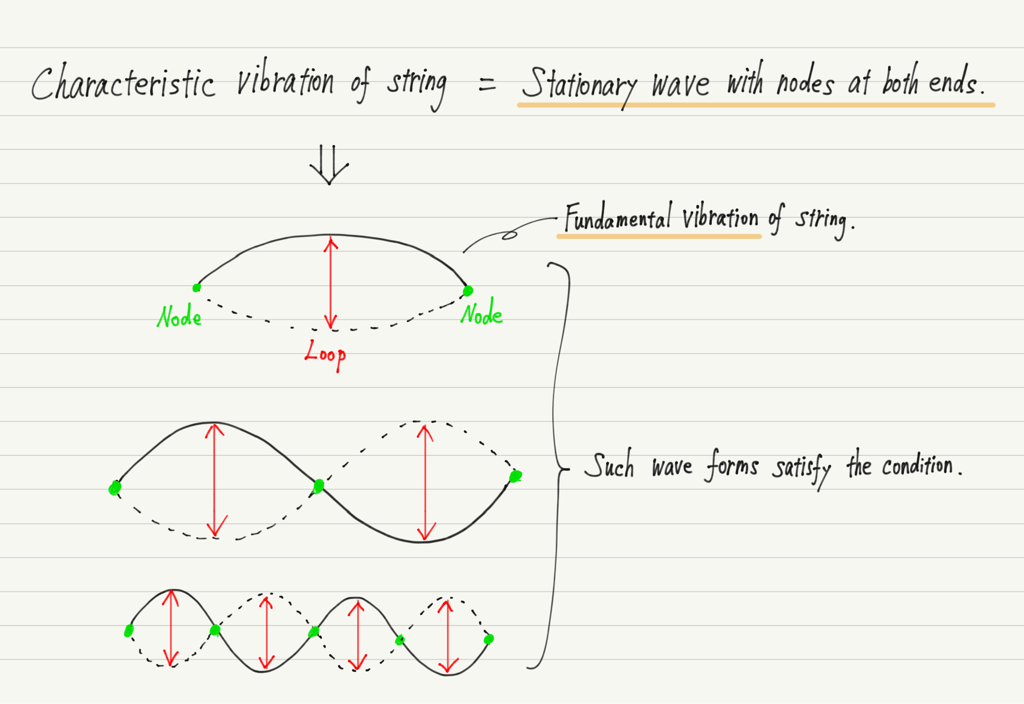There are several possible vibrations as shown in the figure above, and the simplest form of the stationary wave is called the fundamental vibration.
In this case, the fundamental vibration is a stationary wave with only one loop.

Other stationary waves are distinguished by the number of fundamental vibrations, since they have the shape of multiple fundamental vibrations attached to them.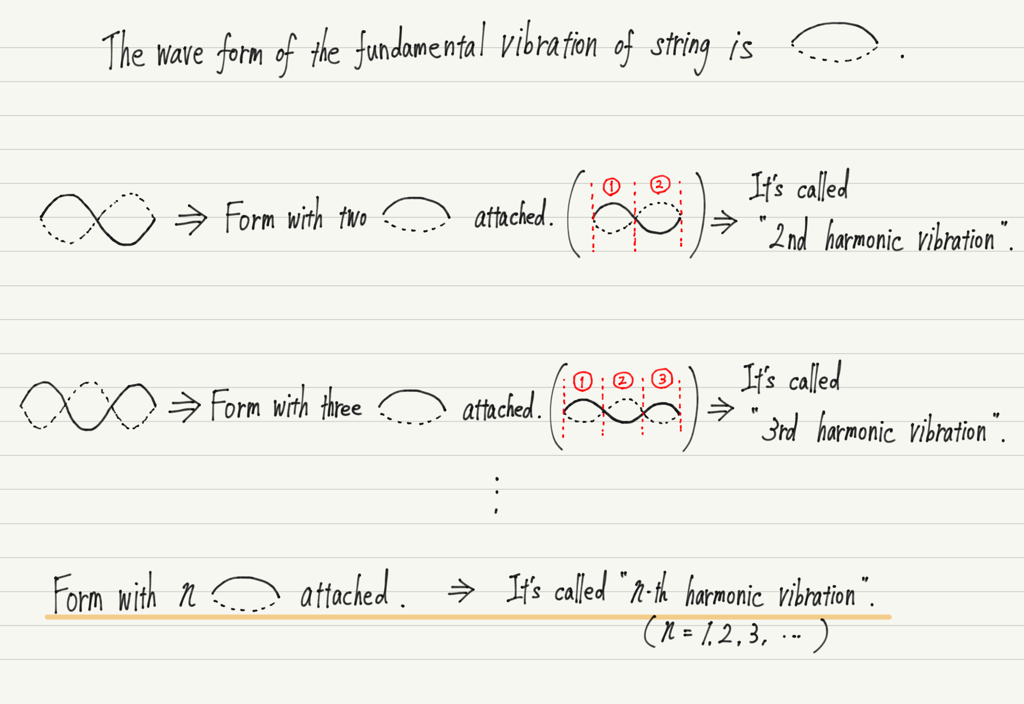In stringed instruments, these vibrations are produced in the strings and mix to produce sound.

## Consideration of characteristic vibrations of string

Let’s find the frequency (eigen frequency) of these vibrations in the string!

Let the length of the string be L, and consider the cases of fundamental, 2nd harmonic and 3rd harmonic vibration.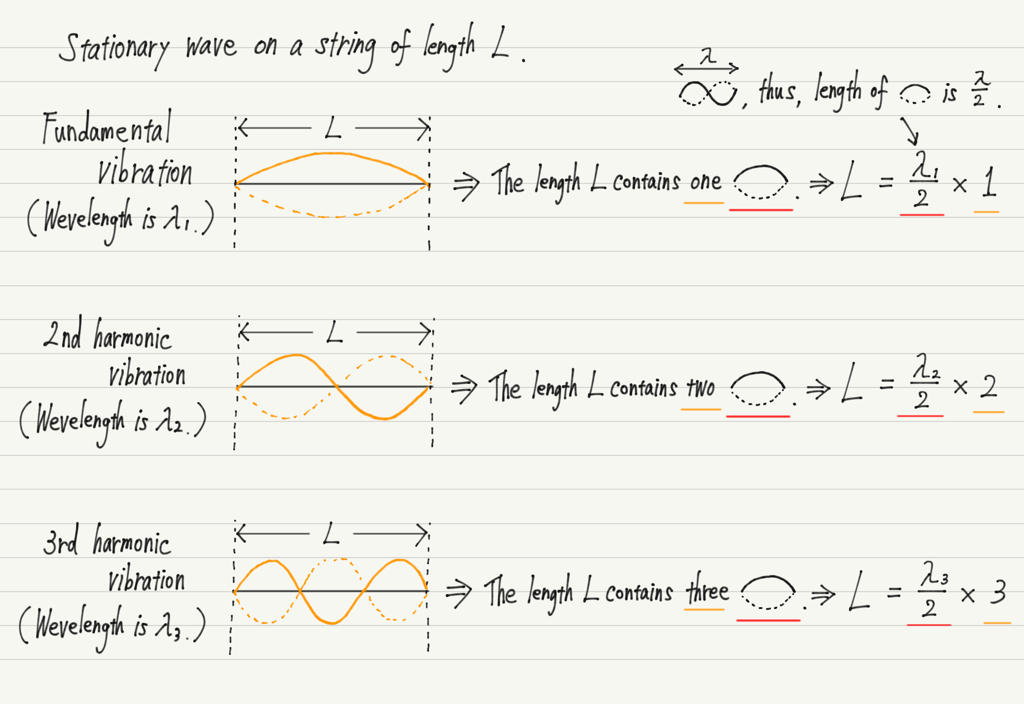Thus, we obtain the equation relating the length of the string to the wavelength.
Now, once we know the wavelength, we can use v=fλ to find the frequency!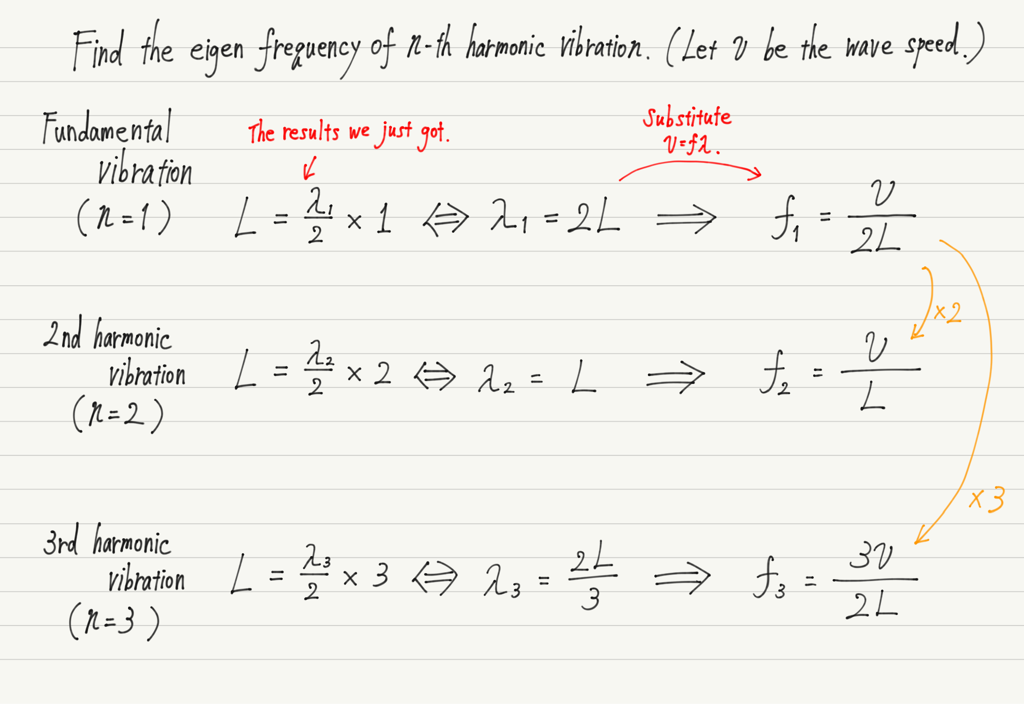These are the eigen frequencies of fundamental, 2nd harmonic and 3rd harmonic vibration.

Looking at this, the eigen frequency of the 2nd harmonic vibration is twice that of the fundamental vibration, and the frequency of the 3rd harmonic vibration is 3 times that of the fundamental vibration.
Is this also the case after the 4th harmonic vibration?

Let’s consider a generalization.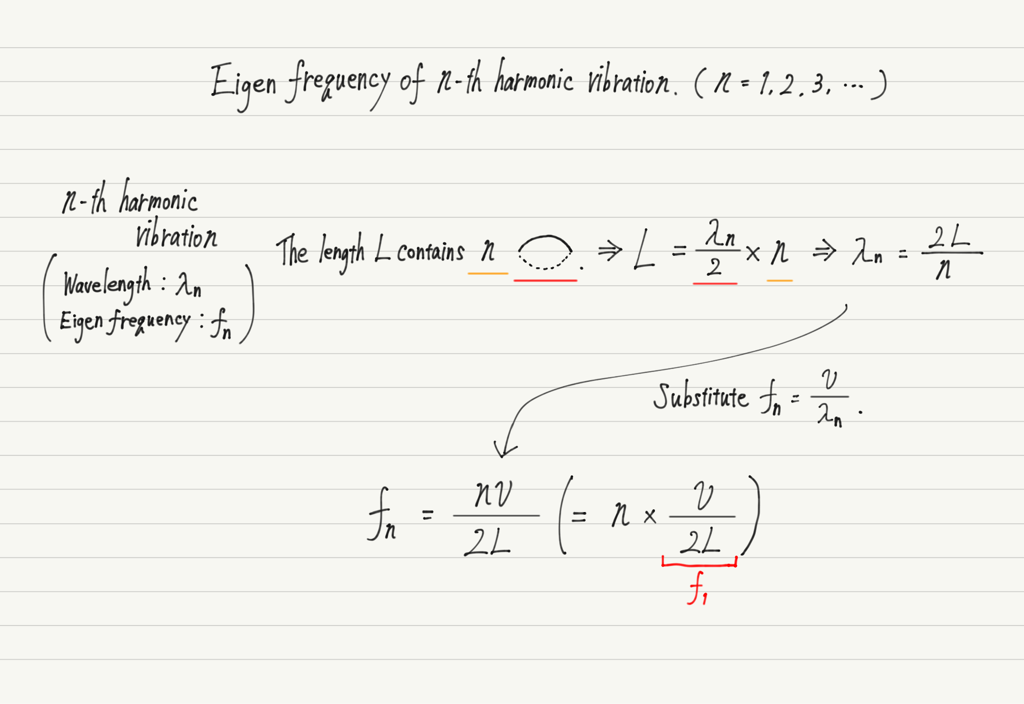This is the eigen frequency of n-th harmonic vibration, which is indeed n times the fundamental vibration.

This eigen frequency equation should be understood, including the process of derivation!

## Strings and Eigen frequency

Now that we have the expression for the eigen frequency of the string, let’s take a closer look.

First, we see that the eigen frequency is proportional to the speed of the wave traveling down the string.
The speed of waves traveling down a string is related to the tension of the string and the mass per unit length.

When tuning the pitch of a stringed instrument, the tension of the string is increased or decreased, and this tension controls the speed of the wave.

The greater the mass of the string, the slower the wave propagates.
The reason the six strings of the guitar are of different thicknesses is that changing the scale requires changing the mass.

Next, the length of the string.
We see that the eigen frequency is inversely proportional to the length of the string.
n other words, the longer the strings, the lower the frequency of vibration and the lower the sound.
Come to think of it, basses have longer strings than guitars!

Conversely, if the strings are shortened, the sound will be higher, and string playing takes advantage of this property.
Of course, we can’t cut the strings while playing, so instead we press down on the strings with our fingers.
The point where the string is pressed is the fixed end, and vibrations are not transmitted beyond the point where the string is pressed.
This is the same as playing a short string.

It’s amazing that so much can be learned about the principles of stringed instruments from just one eigen frequency equation!

## Summary of this lecture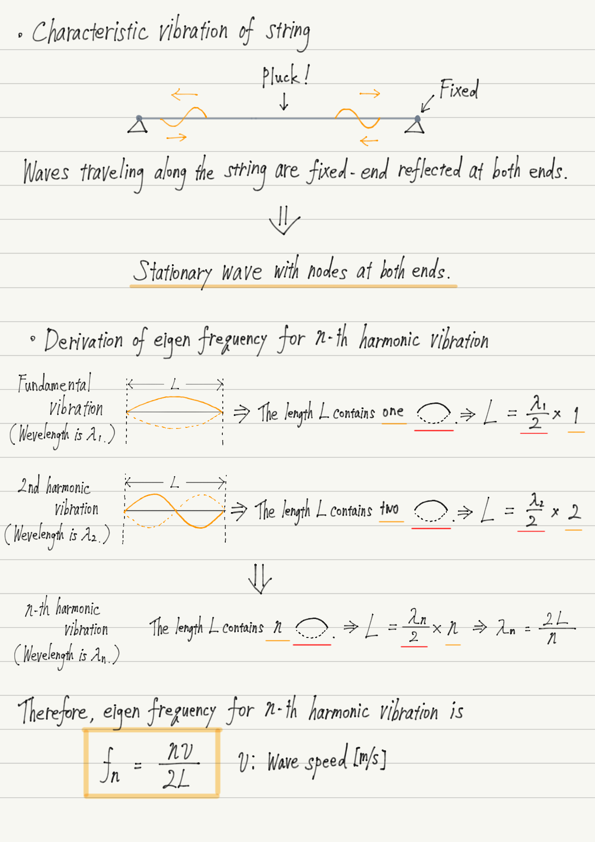## Next Time

Now let’s consider the nature of wind instruments!

https://www.yukimura-physics.com/entry/wave-f19

error: Content is protected !!